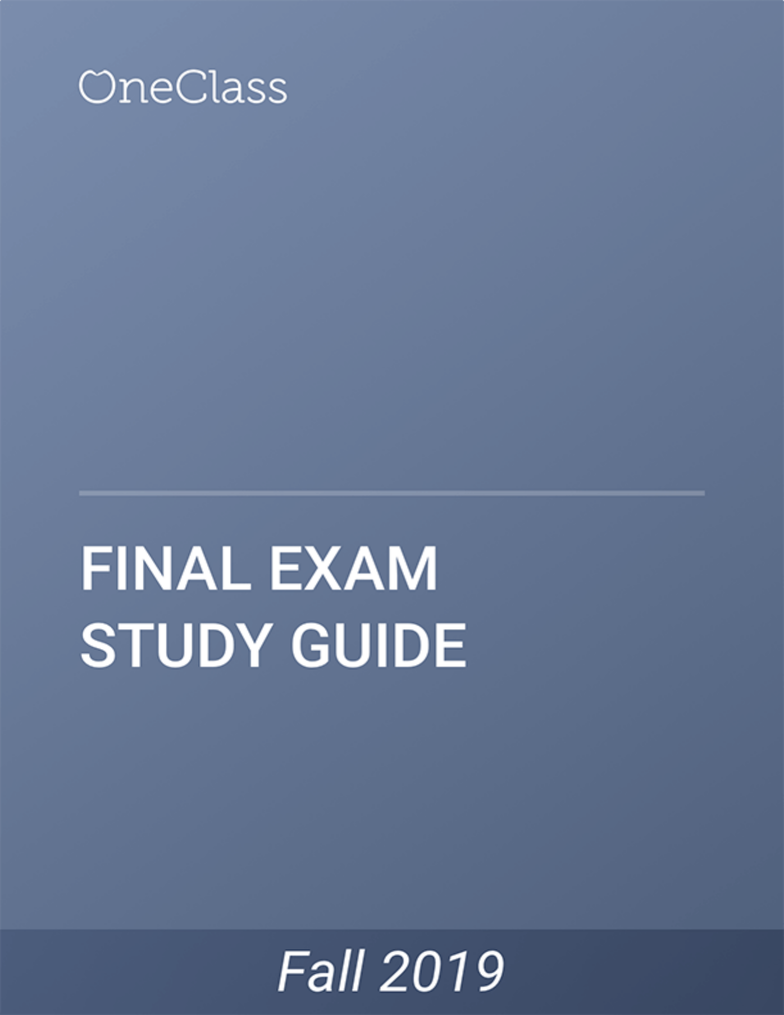# CSCI 2170 Study Guide - Fall 2019, Comprehensive Final Exam Notes - Euclidean Vector, Wireless Access Point, Integer

13 views27 pages
School
Course
ProfessorCSCI 2170
Unlock document

This preview shows pages 1-3 of the document.
Unlock all 27 pages and 3 million more documents.Unlock document

This preview shows pages 1-3 of the document.
Unlock all 27 pages and 3 million more documents.Math 241 Chapter 14 Dr. Justin O. Wyss-Gallifent
§14.1 Double Integrals
1. These can be deﬁned via a Riemann Sum method like in Calculus I but the net result is: We can
deﬁne the double integral of f(x, y)over R, denoted RRRf(x, y)dA to be the signed volume under
the graph of f(x, y) within the region R. The question is how to evaluate these things. First...
2. Defn: An iterated integral is a nested integral. An inner integral may have limits of integration
which include variables further out. We evaluate these by working from the inside out, making
sure we integrate with respect to the correct variable each time.
3. Now then, onto evaluation of RRRf(x, y)dA.
(a) Ris vertically simple if Rmay be described as between the two functions y=bot(x) and
y=top(x) on the interval axb. In this case RR
R
f(x, y)dA =Rb
aRtop
bot f(x, y)dy dx.
(b) Ris horizontally simple if Rmay be described as between the two functions y=lef t(x) and
y=right(x) on the interval cyd. In this case RR
R
f(x, y)dA =Rd
cRright
lef t f(x, y)dx dy.
Consider in both cases that if either the top, bottom, left or right function ever changes then you
will need more than one integral.
4. We can reparametrize (HS to VS or VS to HS) to do an impossible integral like R1
0R1
xe(y2)dy dx.
§14.2 Double Integrals in Polar Coordinates
1. Reminder about how polar coordinates work. Shapes we’ll see a lot include things like r= 2,
r= 3 cos θ,r= 2 sin θ,r= 1 + cos θas well as vertical and horizontal lines which need to be
converted. Don’t forget x=rcos θ,y=rsin θand x2+y2=r2.
2. We describe a region in polar coordinates from the point of view of a person who lives at the
origin. There is a near function r=near(θ) and a far function r=far(θ) between two angles
αθβ. In this case RR
R
f(x, y)dA =Rβ
αRfar
near f(rcos θ, r sin θ)r dr dθ. We’ll see later where
that extra rcomes from. It might help to remember it’s the “Jacobian r”.
3. We can reparametrize (to polar) to do an impossible integral like R1
1R1x2
0sin(x2+y2)dy dx.
§14.4 Triple Integrals
1. Finding a volume analogy is tricky. Instead suppose Dis a solid object in space and at any point
f(x, y, z) is the density around that point. Then we can deﬁne RRR
D
f(x, y, z)dV as the mass of
D. The question is how to evaluate which all depends upon how to best describe D.
2. We have the following:
(a) If Dis the solid between the graphs of z=low(x, y) and z=high(x, y) above the region R
in the xy-plane and if Ris VS then RRR
D
f(x, y, z)dV =Rb
aRtop
bot Rhigh
low f(x, y, z)dz dy dx.
(b) If Dis the solid between the graphs of z=low(x, y) and z=high(x, y) above the region R
in the xy-plane and if Ris HS then RRR
D
f(x, y, z)dV =Rd
cRright
lef t Rhigh
low f(x, y, z)dz dx dy.
find more resources at oneclass.com
find more resources at oneclass.com
Unlock document

This preview shows pages 1-3 of the document.
Unlock all 27 pages and 3 million more documents.§14.5 Triple Integrals in Cylindrical Coordinates
1. Cylindrical coordinates are just polar coordinates plus z. The thing to watch out for is how
equations change. For example:
(a) r= 2 is a cylinder, as are r= 3 cos θand r= 2 sin θ.
(b) The sphere x2+y2+z2= 9 becomes r2+z2= 9.
(c) The cone z=px2+y2becomes z=r.
(d) The plane x= 2 becomes rcos θ= 2 or r= 2 sec θ.
2. If Dis the solid between the graphs of z=low(x, y) and z=high(x, y) above the region Rin the
xy-plane and if Ris polar then we have to convert low and high to polar functions z=low(r, θ)
and z=high(r, θ) in terms of rand/or θand then
RRR
D
f(x, y, z)dV =Rβ
αRfar
near Rhigh
low f(rcos θ, r sin θ, z)r dz dθ dr.
§14.6 Triple Integrals in Spherical Coordinates
1. Describe how spherical coordinates work and make sure to mention the conversions:
(a) x=ρsin φcos θ
(b) y=ρsin φsin θ
(c) z=ρcos φ
(d) x2+y2+z2=ρ2
(e) x2+y2=ρ2sin2φ
2. Equations can change here. For example:
(a) ρ= 2 is a sphere.
(b) The cylinder x2+y2= 4 becomes ρ= 2 csc φ.
(c) φ=π
4is a cone.
(d) The plane z= 3 becomes ρ= 3 sec φ.
3. To describe a solid in spherical we take a range αθβand γφδFrom the point of view
of a person at the origin this describes a “window” looking out. In that window we have a near
function ρ=near(φ, θ) and a far function ρ=far(φ, θ).
4. If Dis described this way then
RRR
D
f(x, y, z)dV =Rβ
αRδ
γRfar
near f(ρsin φcos θ, ρ sin φsin θ, ρ cos φ)ρ2sin φ dρ dφ dθ.
Don’t forget that ρ2sin φ. It’s the “Jacobian” again.
find more resources at oneclass.com
find more resources at oneclass.com
Unlock document

This preview shows pages 1-3 of the document.
Unlock all 27 pages and 3 million more documents.